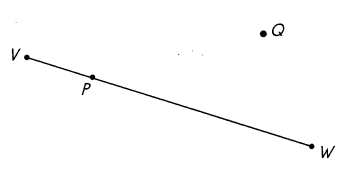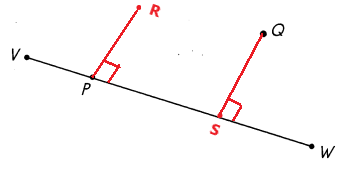# Math in Focus Grade 4 Chapter 10 Practice 1 Answer Key Drawing Perpendicular Line Segments

This handy Math in Focus Grade 4 Workbook Answer Key Chapter 10 Practice 1 Drawing Perpendicular Line Segments detailed solutions for the textbook questions.

## Math in Focus Grade 4 Chapter 10 Practice 1 Answer Key Drawing Perpendicular Line Segments

Use a protractor to draw perpendicular line segments.

Example

Question 1.
Draw a line segment perpendicular to $$\overline{P Q}$$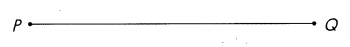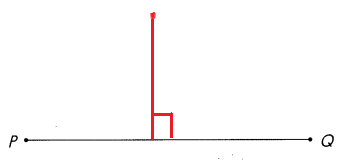Drawn a line segment perpendicular to PQ.

Question 2.
Draw a line segment perpendicular to $$\overline{T U}$$ through point X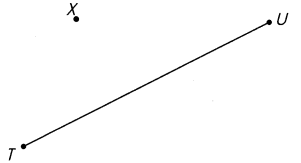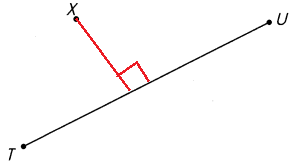Drawn a line segment perpendicular to TU through point X.

Use a drawing triangle to draw perpendicular line segments.

Example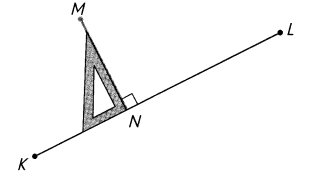Question 3.
Draw a line segment perpendicular to $$\overline{E F}$$.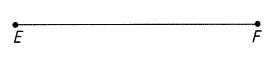Draw a line segment perpendicular to EF.

Question 4.
Draw a line segment perpendicular to $$\overline{C D}$$.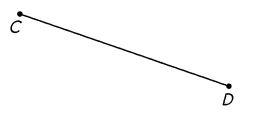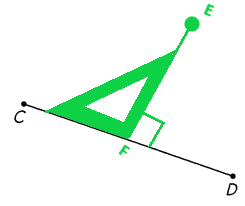Draw a line segment perpendicular to $$\overline{V W}$$ at point P.
Then, draw another line segment perpendicular to $$\overline{V W}$$ through point Q.